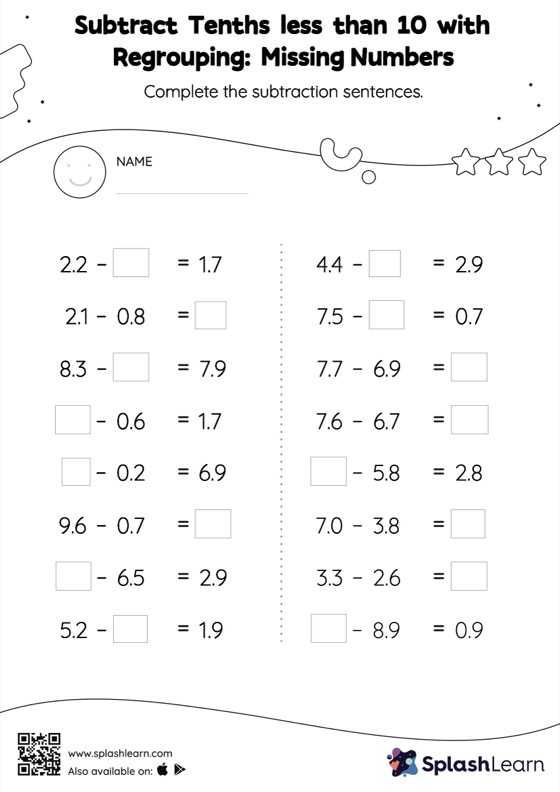# Subtract Tenths less than 10 with Regrouping: Missing Numbers Worksheet

Home > Subtract Tenths less than 10 with Regrouping: Missing NumbersGive your little one some practice building subtraction skills with this subtract tenths less than 10 with regrouping worksheet. While subtracting decimals, students use the relationship between addition and subtraction to find the missing number. They also need to regroup numbers in subtract tenths less than 10 with regrouping worksheet utilizing the relationship between ones, tenth, hundredth, thousandth, etc., To get to the result.